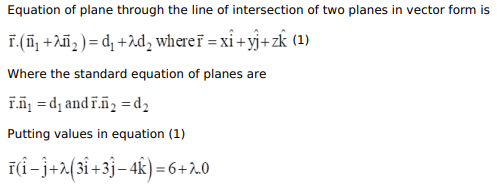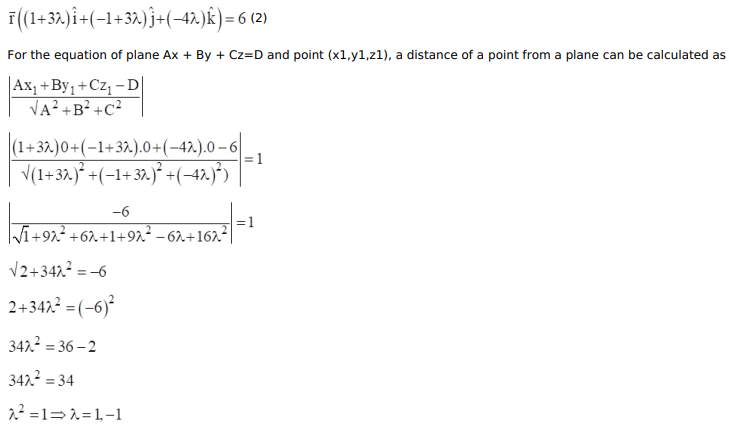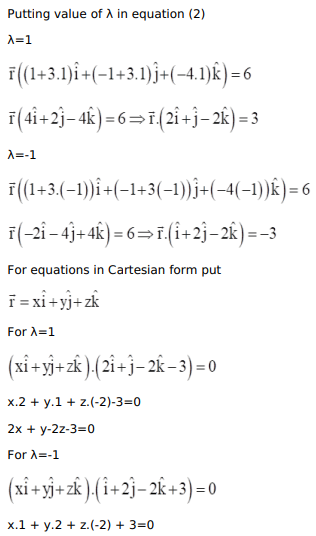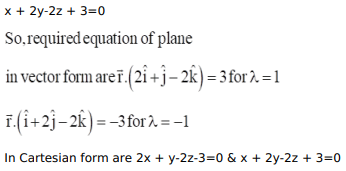# Find the Cartesian and vector equations of the planes through the line of intersection of the planes

Question:

Find the Cartesian and vector equations of the planes through the line of intersection of the planes $\overrightarrow{\mathrm{r}} \cdot(\hat{\mathrm{i}}-\hat{\mathrm{j}})+6=0$ and $\overrightarrow{\mathrm{r}} \cdot(3 \hat{\mathrm{i}}+3 \hat{\mathrm{j}}-4 \hat{\mathrm{k}})=0$, which are at a unit distance from the origin.

Solution: